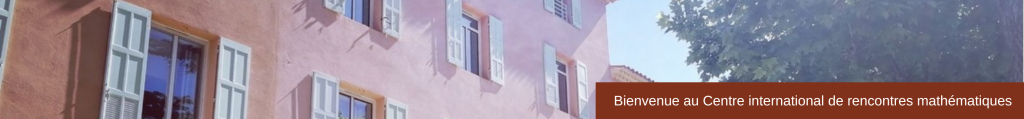CONFERENCE
New Challenges in Mathematical Modelling and Numerical Simulation of Superfluids
June 27 – July 1, 2016
 Research in superfluidity (including Bose-Einstein Condensation, superfluid Helium and supraconductors) has a rapidly growing dynamics in Physics, motivated by applications which may lead to a new technology revolution. In parallel, mathematical studies related to these models (nonlinear Schrödinger equation, Gross-Pitaevskii equation, Ginzburg-Landau equation) have known a dramatic development, associated with rigorous mathematical theories and advanced numerical methods. The goal of this conference is to bring together physicists and mathematicians whose expertise in this field is renowned, thus easing the interdisciplinary communication and allowing some future collaborations. This conference will also be the opportunity to present the results obtained in the framework of the BECASIM ANR project, whose goal is to establish a new state of the art in the field of numerical methods and high performance computing in the simulation of Bose-Einstein Condensation. As the conference takes place during the final year of the project, this will be the opportunity to present the mathematical models, the numerical methods, parallel computing codes, so they can be shared with physicists, and to make plans on future research. Scientific & Organizing Committee Weizhu Bao (National University of Singapore)Rémi Carles (Université de Montpellier)Ionut Danaila (Université de Rouen) Speakers Mauro Antezza (Université de Montpellier) Solitons in a superfluid Fermi gas ﻿Xavier Antoine ﻿(Université de Lorraine) GPELab, an open source Matlab toolbox for the numerical simulation of Gross-Pitaevskii equations Weizhu Bao  (National University of Singapore) Quantized vortex stability and dynamics in superfluidity and superconductivity Carlo Barenghi (Newcastle University) Classical and non-classical flows of superfluids Natalia Berloff (University of Cambridge) Polariton graph simulators Christophe Besse (Université Toulouse 3) High-order numerical schemes for computing the dynamics of nonlinear Schrödinger equation Marc Brachet (ENS Paris) Helicity, topology and Kelvin waves in reconnecting quantum knots Yongyong Cai (Purdue University and Beijing Computational Science Research Center) Ground states and dynamics of spin-orbit-coupled Bose-Einstein condensates Rémi Carles (Université de Montpellier) Time splitting methods and the semi-classical limit Frédéric Chevy (ENS Paris) Counterflowing superfluids Ionut Danaila (Université de Rouen Normandie) An overview of the BECASIM project: open source numerical simulators for the Gross-Pitaevskii equation Anne De Bouard (Ecole polytechnique) Inhomogeneities and temperature effects in Bose-Einstein condensates Qiang Du (Columbia University) Nonlocal models and their numerical discretization Arnaldo Gammal (University of São Paulo) Three-dimensional vortex structures in a rotating dipolar Bose-Einstein condensate Jean-Claude Garreau (PhLAM Lille) Symmetries and dynamics in a quantum-chaotic system Dieter Jaksch (University of Oxford) Plasmonics in layered superconductors Robert Jerrard (University of Toronto) Nearly parallel vortex filaments in the 3d Gunzburg-Landau equations Michikazu Kobayashi (University of Tokyo) Quantum nature and statistical law in quantum turbulence Mathieu Lewin (Université Paris Dauphine) Superfluidity and Bogoliubov theory: rigorous derivation for mean-field many-body systems Philippe Parnaudeau (UPMC) A hybrid code for solving the Gross-Pitaevskii equation Bartosz Protas (McMaster University) On Some Variational Optimization Problems in Classical Fluids and Superfluids Nikolaus Proukakis (Newcastle University) Quantum Fluid Mixtures: Modelling Phase Separation & Dynamics of Atomic Bose-Einstein Condensates Etienne Sandier (Université Paris 12) Young measures for homogenization of phase transition models Didier Smets (UPMC) Leapfrogging for the axisymmetric Gross-Pitaevskii equation Henk Stoof  (Utrecht University) Order parameter fluctuations in the holographic superconductor Qinglin Tang (INRIA Nancy Grand-Est) Numerical methods on simulating dynamics of the nonlinear Schrödinger equation  with rotation and/or nonlocal interactions Mechthild Thalhammer (University of Innsbruck) High-order Magnus integrators for non-autonomous linear evolution equations Makoto Tsubota (Osaka City University) Inhomogeneous quantum turbulence in a channel Hanquan Wang (Yunnan University, China) An efficient splitting Fourier pseudospectral method for Vlasov-Poisson-Fokker-Planck system Yanzhi Zhang (Missouri University) Fractional Schrödinger equa tion: stationary states and dynamics